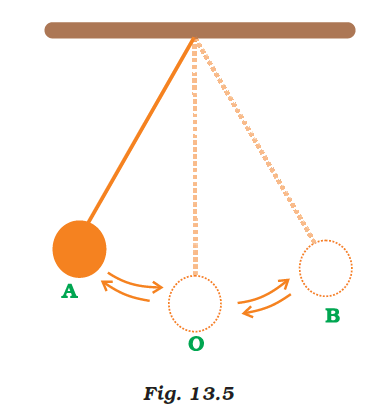# A simple pendulum is oscillating between two points A and B as shown in Figure. Is the motion of the bob uniform or non-uniform?A point mass attached to a light inextensible string and suspended from fixed support is called a simple pendulum. The vertical line passing through the fixed support is the mean position of a simple pendulum.

A uniform motion is created by a simple pendulum oscillating between two points A and B. The motion is uniform because Bob moves at a constant speed throughout the motion.(0)(0)